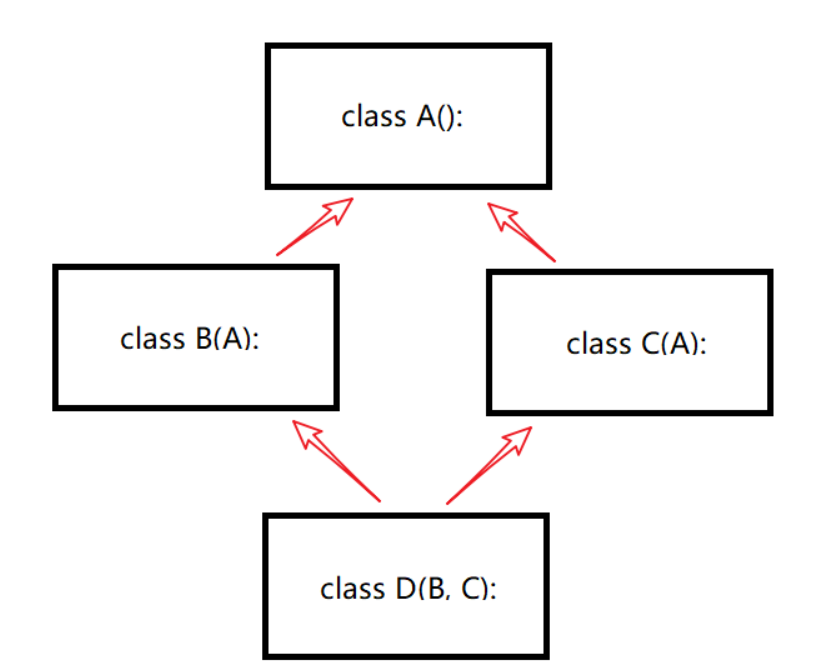### Python中的多重继承

``class SubclassName(BaseClass1, BaseClass2, BaseClass3, ...):    pass``

# 菱形继承• D->B->A->C（深度优先）
• D->B->C->A（广度优先）

``class A():    def __init__(self):        print('init A...')        print('end A...') class B(A):    def __init__(self):        print('init B...')        A.__init__(self)        print('end B...') class C(A):    def __init__(self):        print('init C...')        A.__init__(self)        print('end C...') class D(B, C):    def __init__(self):        print('init D...')        B.__init__(self)        C.__init__(self)        print('end D...') if __name__ == '__main__':    D() ''' ------ Output ------init D...init B...init A...end A...end B...init C...init A...end A...end C...end D...'''``

``class A():    def __init__(self):        print('init A...')        print('end A...') class B(A):    def __init__(self):        print('init B...')        super(B, self).__init__()        print('end B...') class C(A):    def __init__(self):        print('init C...')        super(C, self).__init__()        print('end C...') class D(B, C):    def __init__(self):        print('init D...')        super(D, self).__init__()        print('end D...') if __name__ == '__main__':    D() ''' ------ Output ------init D...init B...init C...init A...end A...end C...end B...end D...'''``

Python类分为两种，一种叫经典类，一种叫新式类。都支持多继承，但继承顺序不同。

• 新式类：从object继承来的类。如class A(object)，采用广度优先搜索的方式继承（即先水平搜索，再向上搜索）。
• 经典类：不从object继承来的类。如class A()，采用深度优先搜索的方式继承（即先深入继承树的左侧，再返回，再找右侧）。

Python 2.x中的类是有经典类和新式类两种，Python 3.x中都是新式类。